# sklearn.datasets.make_classification¶

sklearn.datasets.make_classification(n_samples=100, n_features=20, *, n_informative=2, n_redundant=2, n_repeated=0, n_classes=2, n_clusters_per_class=2, weights=None, flip_y=0.01, class_sep=1.0, hypercube=True, shift=0.0, scale=1.0, shuffle=True, random_state=None)[source]

Generate a random n-class classification problem.

This initially creates clusters of points normally distributed (std=1) about vertices of an n_informative-dimensional hypercube with sides of length 2*class_sep and assigns an equal number of clusters to each class. It introduces interdependence between these features and adds various types of further noise to the data.

Without shuffling, X horizontally stacks features in the following order: the primary n_informative features, followed by n_redundant linear combinations of the informative features, followed by n_repeated duplicates, drawn randomly with replacement from the informative and redundant features. The remaining features are filled with random noise. Thus, without shuffling, all useful features are contained in the columns X[:, :n_informative + n_redundant + n_repeated].

Read more in the User Guide.

Parameters:
n_samplesint, default=100

The number of samples.

n_featuresint, default=20

The total number of features. These comprise n_informative informative features, n_redundant redundant features, n_repeated duplicated features and n_features-n_informative-n_redundant-n_repeated useless features drawn at random.

n_informativeint, default=2

The number of informative features. Each class is composed of a number of gaussian clusters each located around the vertices of a hypercube in a subspace of dimension n_informative. For each cluster, informative features are drawn independently from N(0, 1) and then randomly linearly combined within each cluster in order to add covariance. The clusters are then placed on the vertices of the hypercube.

n_redundantint, default=2

The number of redundant features. These features are generated as random linear combinations of the informative features.

n_repeatedint, default=0

The number of duplicated features, drawn randomly from the informative and the redundant features.

n_classesint, default=2

The number of classes (or labels) of the classification problem.

n_clusters_per_classint, default=2

The number of clusters per class.

weightsarray-like of shape (n_classes,) or (n_classes - 1,), default=None

The proportions of samples assigned to each class. If None, then classes are balanced. Note that if len(weights) == n_classes - 1, then the last class weight is automatically inferred. More than n_samples samples may be returned if the sum of weights exceeds 1. Note that the actual class proportions will not exactly match weights when flip_y isn’t 0.

flip_yfloat, default=0.01

The fraction of samples whose class is assigned randomly. Larger values introduce noise in the labels and make the classification task harder. Note that the default setting flip_y > 0 might lead to less than n_classes in y in some cases.

class_sepfloat, default=1.0

The factor multiplying the hypercube size. Larger values spread out the clusters/classes and make the classification task easier.

hypercubebool, default=True

If True, the clusters are put on the vertices of a hypercube. If False, the clusters are put on the vertices of a random polytope.

shiftfloat, ndarray of shape (n_features,) or None, default=0.0

Shift features by the specified value. If None, then features are shifted by a random value drawn in [-class_sep, class_sep].

scalefloat, ndarray of shape (n_features,) or None, default=1.0

Multiply features by the specified value. If None, then features are scaled by a random value drawn in [1, 100]. Note that scaling happens after shifting.

shufflebool, default=True

Shuffle the samples and the features.

random_stateint, RandomState instance or None, default=None

Determines random number generation for dataset creation. Pass an int for reproducible output across multiple function calls. See Glossary.

Returns:
Xndarray of shape (n_samples, n_features)

The generated samples.

yndarray of shape (n_samples,)

The integer labels for class membership of each sample.

make_blobs

Simplified variant.

make_multilabel_classification

Unrelated generator for multilabel tasks.

Notes

The algorithm is adapted from Guyon  and was designed to generate the “Madelon” dataset.

References



I. Guyon, “Design of experiments for the NIPS 2003 variable selection benchmark”, 2003.

## Examples using sklearn.datasets.make_classification¶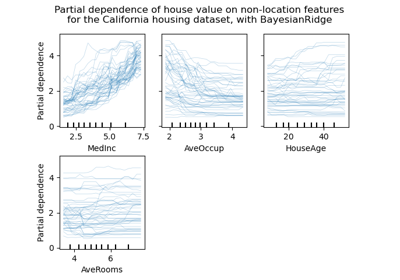Release Highlights for scikit-learn 0.24

Release Highlights for scikit-learn 0.24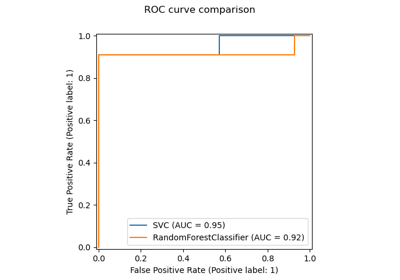Release Highlights for scikit-learn 0.22

Release Highlights for scikit-learn 0.22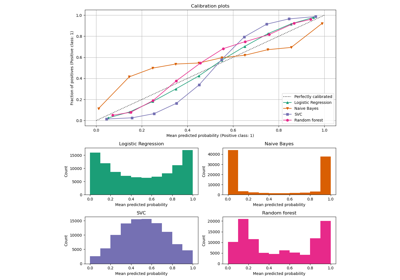Comparison of Calibration of Classifiers

Comparison of Calibration of Classifiers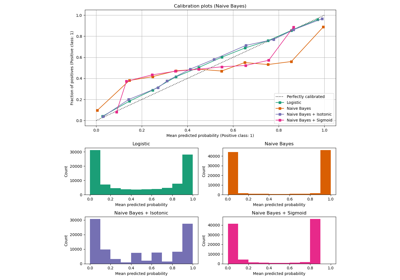Probability Calibration curves

Probability Calibration curves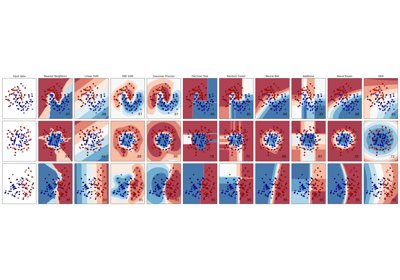Classifier comparison

Classifier comparisonPlot randomly generated classification dataset

Plot randomly generated classification dataset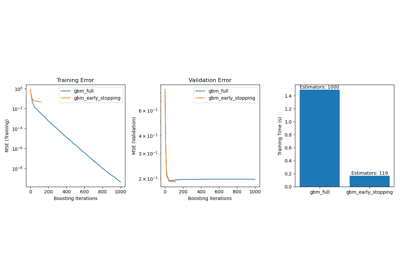Early stopping of Gradient Boosting

Early stopping of Gradient Boosting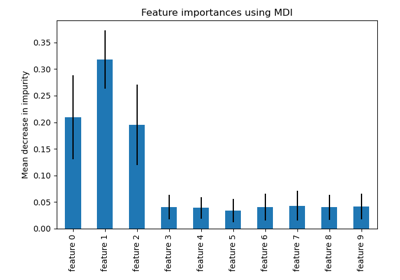Feature importances with a forest of trees

Feature importances with a forest of trees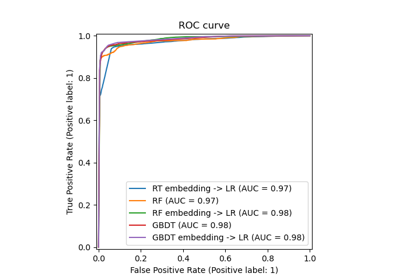Feature transformations with ensembles of trees

Feature transformations with ensembles of trees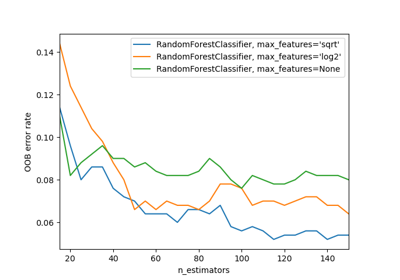OOB Errors for Random Forests

OOB Errors for Random ForestsPipeline ANOVA SVM

Pipeline ANOVA SVM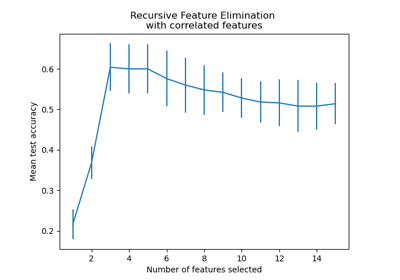Recursive feature elimination with cross-validation

Recursive feature elimination with cross-validation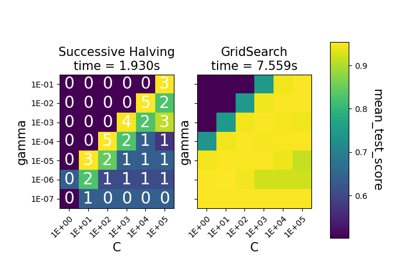Comparison between grid search and successive halving

Comparison between grid search and successive halving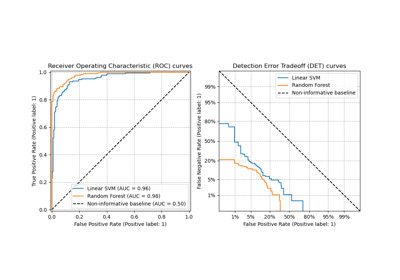Detection error tradeoff (DET) curve

Detection error tradeoff (DET) curve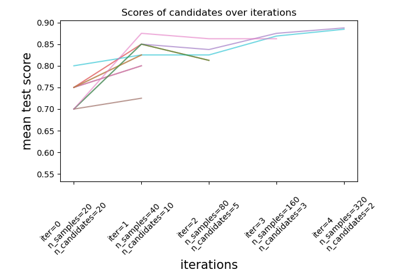Successive Halving Iterations

Successive Halving Iterations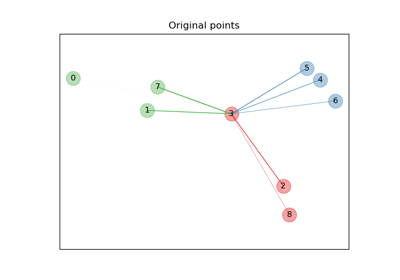Neighborhood Components Analysis Illustration

Neighborhood Components Analysis Illustration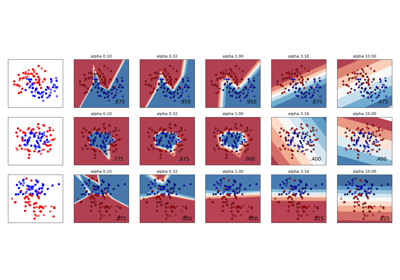Varying regularization in Multi-layer Perceptron

Varying regularization in Multi-layer Perceptron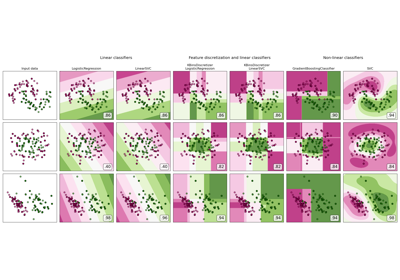Feature discretization

Feature discretization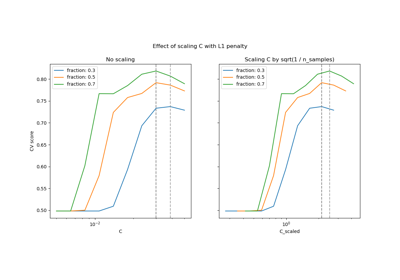Scaling the regularization parameter for SVCs

Scaling the regularization parameter for SVCs